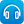Close message Scootle will be undergoing maintenance between 17:00 to 19:00 on 25 September 2023. You may experience intermittent connection during this time. We apologize for any inconvenience caused.

# Search results

## Refine by topic

Main topic Specific topic Related topic
Listed under:  Indices### Numbers Count: What are factors?

What are factors? Watch as the jelly babies in this clip show you! What are the factors of 12? How many factors does the number 11 have? Try explaining to a friend what a prime number is.### reSolve: Algebra: Sums & Differences of Squares

This sequence of three lessons explores sums and differences of two squares. Students are introduced to the historical context of using lookup tables for multiplications and challenged to investigate and generalise the underlying process using algebraic means. In subsequent lessons students use visual and algebraic methods ...### Understanding exponents: 2

This resource is a short video presentation, with audio commentary, in which the meaning of exponents or powers of a number is explained. In the numerical example used the presenter explains the difference between evaluating the power of a number and the product of two numbers.### Catalyst: Prime numbers and unbreakable codes

Imagine if anyone was able to read all our secret, encrypted messages and information. Watch and find out how scientists at the Australian National University are developing a new encryption system using quantum physics and quantum computing.### Catalyst: Small scale measurements

What units of measurements do we use to describe incredibly small things like blood cells and atoms? Watch as you are taken on a journey to explain the different units of measurement that we use to describe the very small.### Self Improvement Wednesday: The beauty of prime numbers

A prime number is a number that only has two factors: one and itself. Listen to Adam Spencer and Richard Glover discussing prime numbers. They cover how we define these numbers and how and why prime numbers are widely used in internet encryption.### Patterns, primes and Pascal's Triangle

Are you intrigued by patterns? Check out Vi Hart as she explains how to visualise patterns in prime numbers, using Ulam's Spiral. Watch as Vi creates patterns, using Pascal's Triangle to explore relationships in number. See what happens when she circles the odd numbers. What rule does she use to create the final pattern?### TIMES Module 34: Number and Algebra: quadratic equations - teacher guide

This is a 19-page guide for teachers. It introduces quadratic equations and methods for solving them.### Algebra four

This is an interactive game for two students in which they solve algebraic equations, similar to 'Connect four'. The players can choose from problems that are one- or two-step, quadratic, have distributive properties or have variables on both sides, and more than one problem type can be chosen. The length of time each player ...### Catalyst: Graham's number

If you were asked what the biggest number you can think of is, what would you say? Infinity? Well, what about the biggest finite number you can think of? Mathematician Ron Graham came across such a gigantic number in his research that, to capture its massive size, he and his colleagues needed to come up with new methods ...### Laptop wrap: Expanding on algebra

Students make a presentation on the index laws, investigate the visual representation of the binomial expansions and design an acronym to help recall the special products.### reSolve: Prime factorisation

This sequence of four lessons explores prime factorisation. Students solve a puzzle using factor strings, play a dice game to learn about prime numbers, develop a method for finding all of the factors of a number, and engage in an investigation of highest common factors and lowest common multiples of two numbers, and how ...### reSolve: Pythagoras' Theorem - Bent bamboo

This lesson challenges students to use Pythagoras' Theorem to solve a problem from an ancient Chinese text. They make physical models of the problem and use this to construct a graph. They use algebra skills associated with binomial expansions and simplification of fractions to show that the general solution given in the ...### reSolve: Algebra: Think of a number- Binomial Equations

This lesson engages students in investigating a 'think of a number' game and then model it visually and algebraically. This develops skills in algebraic operations including expanding, factorising and collecting like terms. Students investigate whether the game will work for any number and are challenged to generate the ...### Secondary mathematics: using real data

These seven learning activities, which focus on the use of 'real data' using a variety of tools (software) and devices (hardware), illustrate the ways in which content, pedagogy and technology can be successfully and effectively integrated in order to promote learning. In the activities, teachers use the three content strands ...